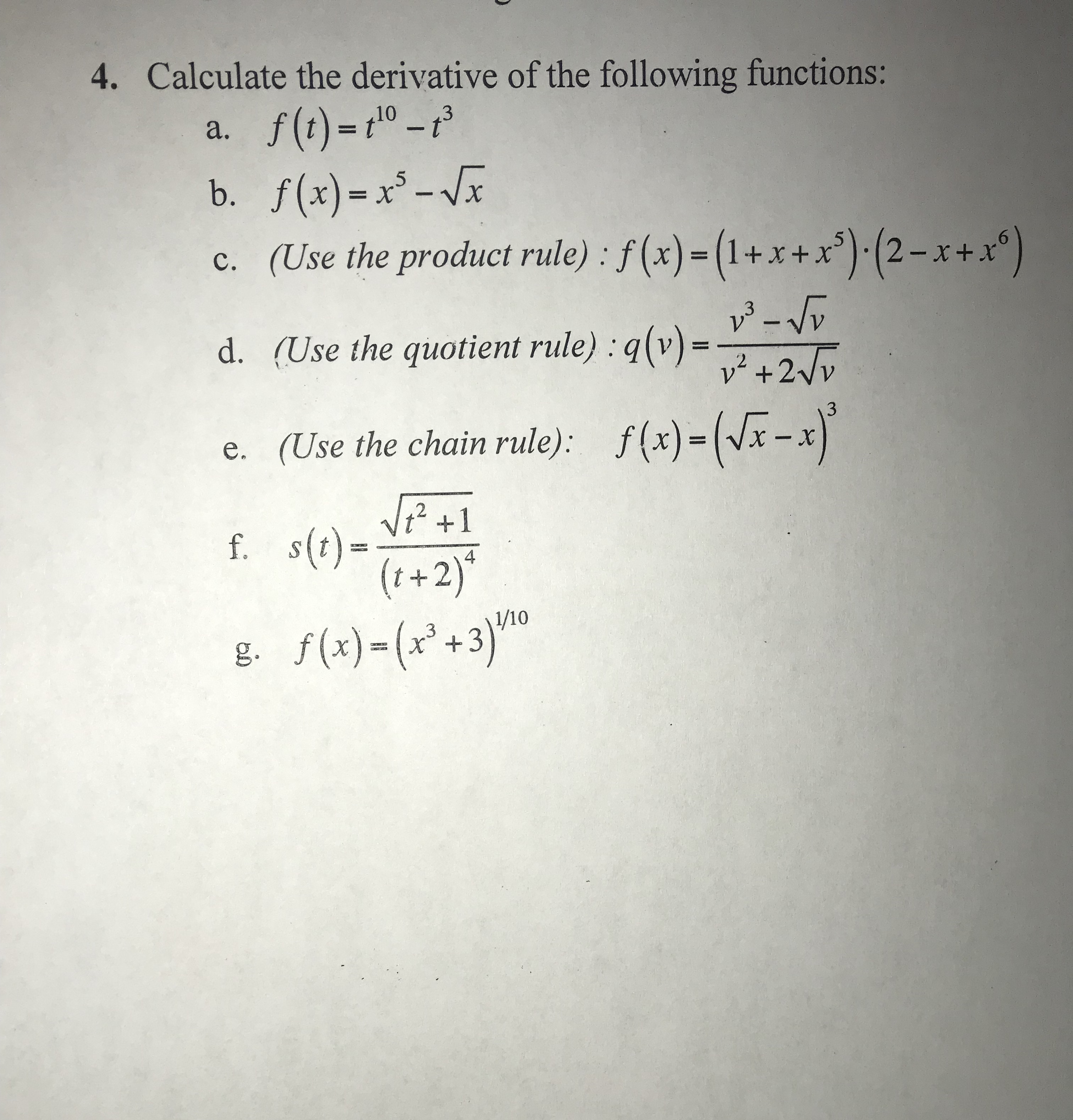# 4. Calculate the derivative of the following functions b. f(x)--x (Use the product rule): f(x)=(1+x+x").(2-x+x" (Use the quotient rule) : q(v)= (Use the chain rule): f(x)=( x-x s(t)= c. d. e. 4 t+2 g. f(x)-(x+3

Question

I need a help with this sample problem. Thank you.help_outlineImage Transcriptionclose4. Calculate the derivative of the following functions b. f(x)--x (Use the product rule): f(x)=(1+x+x").(2-x+x" (Use the quotient rule) : q(v)= (Use the chain rule): f(x)=( x-x s(t)= c. d. e. 4 t+2 g. f(x)-(x+3 fullscreen